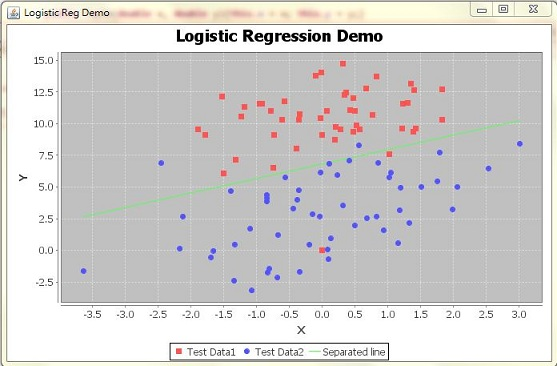2012年11月27日 星期二

[ JLRToolkit ] Logistic Regression Toolkit - Usage tutorial

Preface:

Data format:

Usage Code Example:

- testSet.txt
-0.017612 14.053064 0
-1.395634 4.662541 1
-0.752157 6.538620 0
-1.322371 7.152853 0
0.423363 11.054677 0
0.406704 7.067335 1
0.667394 12.741452 0
-2.460150 6.866805 1
...(紅點為 label=0 的集合; 藍點為 label=1 的集合

- testSet2.txt
1 -0.017612 14.053064 0
1 -1.395634 4.662541 1
1 -0.752157 6.538620 0
1 -1.322371 7.152853 0
1 0.423363 11.054677 0
1 0.406704 7.067335 1
...

1. Utils.SEP_CHAR="\t"// 設定 separator char = Tab
2. Train train = new Train(0.001150); // 設定 ALPHA=0.001; Loop iteration=150
3. train.start(new File("testSet2.txt")); // 對 file=testSet2.txt 進行 training.
4. train.saveModel(new File("Test.model")); // 將 Training 完得到的 weights 矩陣存到 Test.model 檔案中.

[Info] Total 100 records; Feature size=3; Label size=2...
[Info] Default label=1...
[Info] Label=0:[-1.65, -0.12, 0.35]...
[Info] Label=1:[3.53, 0.81, -0.53]...
[Info] Training done! (0 sec)

3.53 + 0.81X + -0.53Y = t

3.53 + 0.81X + -0.53Y = 0
Y = (3.53 + 0.81X) / 0.531. Predict predict = new Predict(new File("Test.model")); // 載入 Model=Test.model
4. predict.start(new File("testSet2.txt"), new File("testPredict.txt")); // 對 "testSet2.txt" 進行 prediction, 並輸出結果到 "testPredict.txt"

[Info] Total 2 labels; Default label=1...
[Info] Total predict 100 records...

Console Model Usage:Supplement:
[ ML In Action ] Predicting numeric values : regression - Linear regression (1)
[ ML In Action ] Predicting numeric values : regression - Linear regression (2)
[ ML In Action ] Predicting numeric values : regression - Linear regression (3)

2012年11月26日 星期一

[ Java 文章收集 ] Java 產生不重覆亂數

Preface:

1. public static int[] GenerateNonDuplicateRan1(int range)
2. {
3.     Random rand = new Random();
4.     int rdm[] = new int[range];
5.     List holeList = new ArrayList();
6.     for(int i=0; i
7.
8.     for(int i=0; i
9.     {
10.         int pv=-1;
11.         do{
12.             pv = (int)(rand.nextDouble()*range);
13.         }while(!holeList.remove(Integer.valueOf(pv)));
14.         rdm[i] = pv;
15.     }
16.     return rdm;
17. }

Collection移出法:

1. 產生一個ArrayList，並且利用for loop塞值進去，你想要產生0~99的亂數，就丟100進去，他就會依序把0~99塞到ArrayList裡面.
2. 用Collection的remove method，隨機的取index，並且移出，直到 ArrayList 的size = 0。因為本來在ArrayList裡面的數字就沒有重複(用for loop塞的)，所以隨機取出的值也不會重複!
3. 最後一個部份就是去呼叫上面的方法並且宣告一個array來接收上面產生的亂數

1. public static int[] GenerateNonDuplicateRan2(int range)
2. {
3.     Random rand = new Random();
4.     int rdm[] = new int[range];
5.     List holeList = new ArrayList();
6.     for(int i=0; i
7.     int cnt=0;
8.     while(holeList.size()>0)
9.     {
10.         int pv = holeList.remove(rand.nextInt(holeList.size()));
11.         rdm[cnt++] = pv;
12.     }
13.     return rdm;
14. }
HashSet 的暴力比較法:

1. public static int[] GenerateNonDuplicateRan3(int range)
2. {
3.     int rdm[] = new int[range];
4.     Random rand = new Random();
5.     HashSet set = new HashSet();
6.     for(int i=0; i
7.     {
8.         int pv = -1;
9.         do
10.         {
11.             pv = rand.nextInt(range);
13.         rdm[i] = pv;
14.     }
15.     return rdm;
16. }

1. public static void main(String args[])
2. {
3.     int range = 1000;
4.     long st = System.currentTimeMillis();
5.     int[] rdm = Rdm.GenerateNonDuplicateRan1(range);
6.     System.out.printf("\t[Info] GenerateNonDuplicateRan1 with Range=%d:\n", range);
7.     long ut = System.currentTimeMillis()-st;
8.     for(int i:rdm) System.out.printf("%d ", i);
9.     System.out.println();
10.     System.out.printf("\t[Info] Spending time=%d ms(%d)!\n\n", ut, rdm.length);
11.
12.     st = System.currentTimeMillis();
13.     rdm = Rdm.GenerateNonDuplicateRan2(range);
14.     System.out.printf("\t[Info] GenerateNonDuplicateRan2 with Range=%d:\n", range);
15.     ut = System.currentTimeMillis()-st;
16.     for(int i:rdm) System.out.printf("%d ", i);
17.     System.out.println();
18.     System.out.printf("\t[Info] Spending time=%d ms!(%d)\n\n", ut, rdm.length);
19.
20.     st = System.currentTimeMillis();
21.     rdm = Rdm.GenerateNonDuplicateRan3(range);
22.     System.out.printf("\t[Info] GenerateNonDuplicateRan3 with Range=%d:\n", range);
23.     ut = System.currentTimeMillis()-st;
24.     for(int i:rdm) System.out.printf("%d ", i);
25.     System.out.println();
26.     System.out.printf("\t[Info] Spending time=%d ms!(%d)\n\n", ut, rdm.length);
27.
28. }

[Info] GenerateNonDuplicateRan1 with Range=1000:
864 435 238 ...
[Info] Spending time=38 ms(1000)!

[Info] GenerateNonDuplicateRan2 with Range=1000:
406 821 595 ...
[Info] Spending time=
1 ms!(1000)

[Info] GenerateNonDuplicateRan3 with Range=1000:
152 959 751 ...
[Info] Spending time=5 ms!(1000)

[ Python 常見問題 ] How to get symbolic link target in Python?

Source From  Here Question How do I extract the target path from a  symbolic link ? HowTo The problem with  os .readlink()  is it will onl...